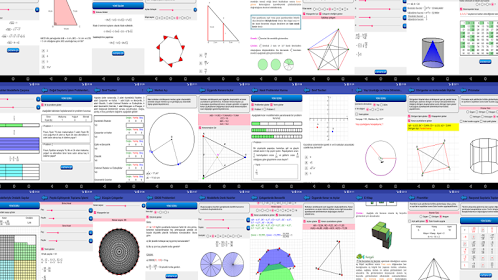# Dynamic StatisticsEveryone
14
This app uses applets to make statistics visual. It includes demonstrations, simulations, games, dynamic calculations and notes about the subjects.

App contents:

* Descriptive statistics
Robustness to outliers
Box plot
Histogram
Stem and leaf plot
Chebyshev’s inequality

* Probability distributions
Binomial distribution
Hypergeometric distribution
Negative binomial distribution
Poisson distribution
Normal distribution
Areas under standard normal distribution
Standard normal distribution table
Areas under Student’s t distribution
Student’s t distribution table
Standard normal and t distribution
Chi-square distribution table
F distribution table

* Probability and simulation
Rock-paper-scissors game
Simulating Monty Hall problem
The birthday problem
Simulating the birthday problem
Simulated coin tossing
Simulated die rolling
Monte Carlo estimate for Pi
The chaos game
The Galton board
The Law of Large Numbers
Central Limit Theorem
k-Means Clustering

* Statistical inference
Sampling distribution of the mean when population is normal and variance known
Sampling distribution of the mean when population is normal and variance unknown
Confidence intervals for the mean and interpretation of confidence level
Significance level and p-value
Correlation coefficient
Linear regression
One way analysis of variance
Collapse

Review Policy
4.5
14 total
5
4
3
2
1

## What's New

* Minimum Spanning Tree applet is added.
Collapse

Updated
September 13, 2018
Size
2.4M
Installs
1,000+
Current Version
1.0.2
Requires Android
4.0 and up
Content Rating
Everyone
Permissions
Offered By
Matletik Yazılım ve Bilişim Ltd. Şti.
Developer
İstanbul Teknokent Ar-Gem Binası, Üniversite Mah, Sarıgül Sokak. No:37/1, 34320-Avcılar, İstanbul